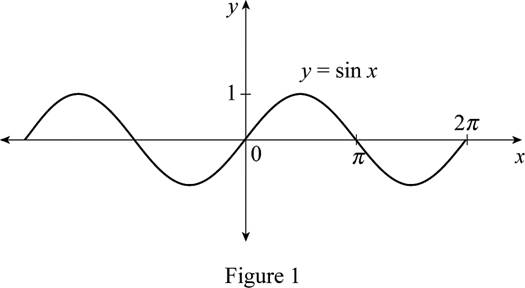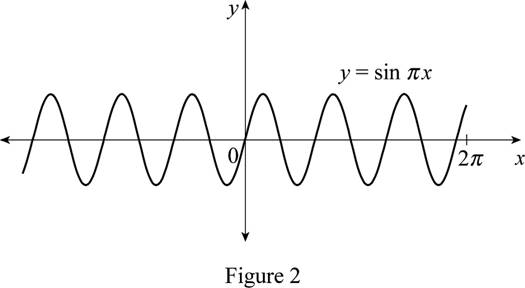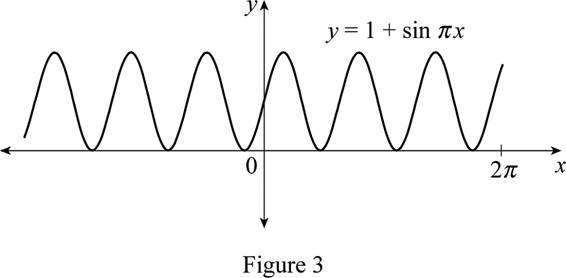# The function y = 1 + sin π x by hand without plotting any points.### Single Variable Calculus: Concepts...

4th Edition
James Stewart
Publisher: Cengage Learning
ISBN: 9781337687805### Single Variable Calculus: Concepts...

4th Edition
James Stewart
Publisher: Cengage Learning
ISBN: 9781337687805

#### Solutions

Chapter 1.3, Problem 16E
To determine

## To sketch: The function y=1+sinπx by hand without plotting any points.

Expert Solution

### Explanation of Solution

The standard graph of the function y=sinx is roughly drawn and shown below in Figure 1.Draw the graph of y=sinπx by shrinking the graph of y=sinx horizontally by a factor of π.

Use online graphing calculator to sketch the graph of y=sinπx as shown below in Figure 2.From Figure 2, it is observed that Figure 1 is compressed horizontally by a factor of π.

To draw the graph of y=1+sinπx, shift the graph y=sinπx by 1 unit upward.

Use online graphing calculator to sketch the graph of y=1+sinπx as shown below in Figure 3.From Figure 3, it is observed that the graph of the function y=1+sinπx lies above the x-axis.

### Have a homework question?

Subscribe to bartleby learn! Ask subject matter experts 30 homework questions each month. Plus, you’ll have access to millions of step-by-step textbook answers!